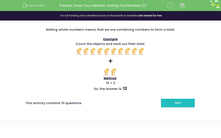### Comprehensive & curriculum aligned

In this worksheet, students count and add the numbers in the range 10-20.Key stage:  KS 1

Curriculum topic:   Number: Addition and Subtraction

Curriculum subtopic:   Solve Add/Subtract Problems to 100

Difficulty level:#### Worksheet Overview

Adding whole numbers means that we are combining numbers to form a total.

Example

Count the objects and work out their total.+Method

10 + 2